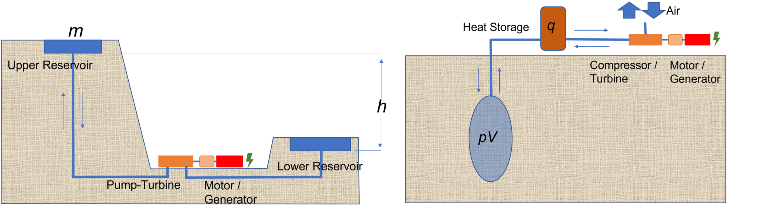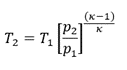## Pumped Hydro and Compressed AirPumped hydro storage and compressed air storage store electricity in the form of potential energy.

## Pumped Hydro Storage

In pumped storage, a certain volume of water with the mass m is pumped into a higher storage basin (upper basin). The amount of energy required for this is m . g . h , where g is the acceleration due to gravity and h is the difference in height between the lower and upper basins. If electricity is required, then water from the upper basin is fed into the lower basin via a turbine. The turbine drives a generator and produces electricity.

Pumped storage can only be built in mountainous regions because of the required height difference and requires large areas for the storage lakes. The expansion of pumped storage is therefore limited.

## Compressed Air Energy Storage - CAES

In compressed air energy storage, air is compressed and stored in cavities. To provide sufficiently large cavities for storage, rock caverns can be built or salt caverns can be excavated. Storage under high pressures in salt caverns is a mature technology and has been used for natural gas storage for more than 50 years.

The energy, as the name compressed air storage implies, is stored as pressure in the compressed air. This appears to be very simple, as it happens, for example, very vividly in an inflated balloon.

## Thermodynamic aspects of compressed air storage

The amount of stored energy can be expressed as the product of the storage volume and the pressure for both pumped storage and compressed air storage.

m.g.Δh = ρ.V.g.Δh = V.Δp

(m is the mass of the water volume, g the acceleration due to gravity, h the height difference, ρ means the density of the water and p stands for the pressure).

For pumped storage, gravity and head provide the storage energy. However, this is not the case for compressed air storage. The compressed air must provide the work for expansion from within itself, and it can only do this if it cools down.

Conversely, the air heats up during compression, as everyone knows from inflating a bicycle tire.

This often undesirable behavior of gases is based on the 1st law of thermodynamics (law of conservation of energy). This reads:

ΔU=Δw + Δq

The internal energy U of a gas is the sum of heat and work. This also describes the equivalence of heat and work. The internal energy cannot be defined as an absolute value, therefore only changes of one quantity are often given in thermodynamics.

An equation of state for a gas describes the relationship between the pressure, the volume and the temperature. For an ideal gas this is:

pV = nRT

Here p is the pressure, V the volume, n the amount of substance, R the general gas constant and T the temperature. The left side of the equation has the dimension of energy as work, the right side as heat. Thus, this equation of state also expresses the equality of work and heat. If one heats the gas so its pressure and its volume change, on the other hand the temperature changes with a pressure or volume change.

If no heat is added to or removed from a gas during compression or expansion, this change of state is called adiabatic. The temperature change occurring here is not linearly dependent on the pressure but:Here κ = cp/cv and cp and cv are the heat capacities of a gas at constant pressure and at constant volume.For a diatomic gas, such as air, κ = 1.4.

If work is to be done with a gas, as in the case of expansion in a turbine, then no energy or heat is added to the gas, which would not make sense either, because energy is to be generated. For this adiabatic (isentropic) expansion, the temperature change results from the above equation.

The temperature changes during compression or expansion of gases can be considerable. For example, for pressure changes of 150 bar, the temperature change is more than 500° Celsius. This is a major disadvantage of compressed air storage. This is to be compensated, as shown in the figure at the very top, by storing the heat. The heat generated during compression is to be returned to the process during expansion.

However, the extraction of work from heat results in a low efficiency of storage. The efficiency in the expansion of a gas is the ratio of the useful work that can be extracted to the total energy. For adiabatic expansion, the efficiency is the ratio of the temperature after expansion T2 and the initial temperature T1 (absolute temperatures in Kelvin).

The volume work w is:

w = c(T2 - T1)

The heat q2, which shall be converted to work is:

q2 = cv T2

Since with adiabatic expansion, no heat is added to or removed from the system, the efficiency is:

η = (-w + q2) / q2

= (- (T2 - T1) +T2) / T2

The heat q2, which is to be converted into work, is retained and is therefore in the numerator of the equation. The heat capacity cv is reduced and

η = T/ T1

For an initial temperature of 500 °C and a temperature after expansion of 20 °C, the following is obtained

η = (273.15+20) / (273.15+500) = 0,38

This is approximately the efficiency that could theoretically be achieved with conventional compressed air storage. However, since complete expansion in the salt or rock caverns is not possible because the temperature would drop far below freezing point and thus damage the rock, the actual efficiency is significantly lower. Simulations, while adhering to the technically permissible boundary conditions, yield efficiencies below 10%.

These  limitations can be avoided by aplication of the erneo technology.